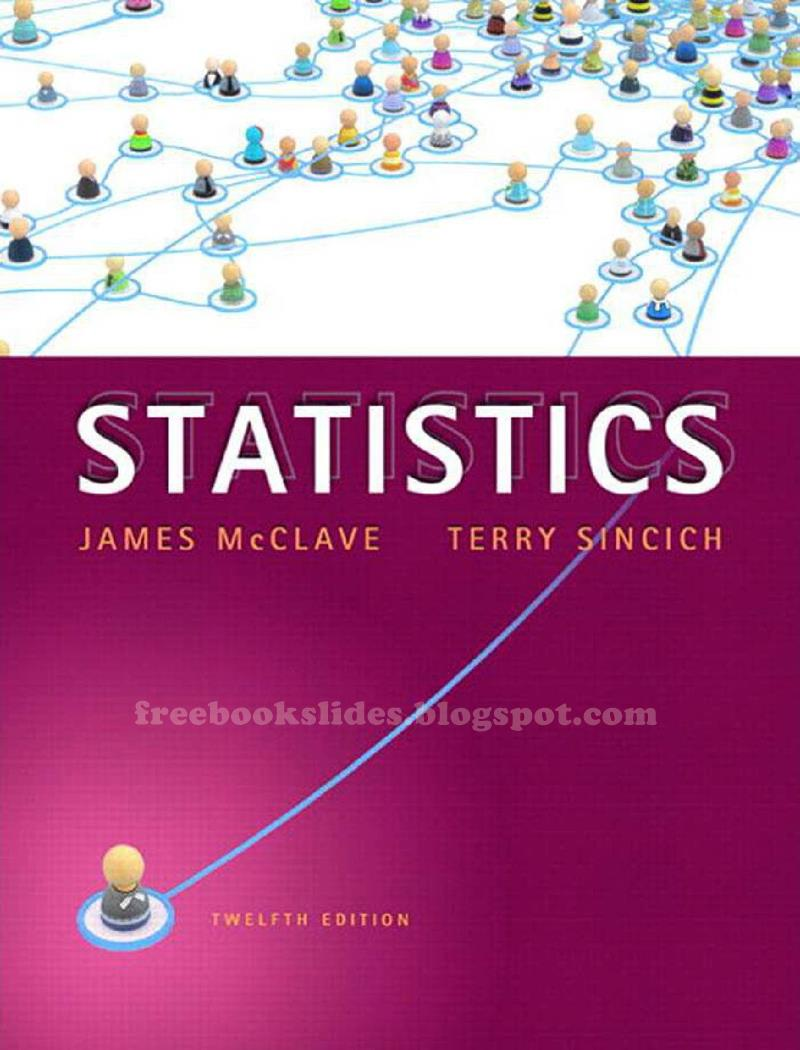### Ebook Statistics 12e by McClave and SincichStatistics
12th Edition
by James T. McClave • Terry Sincich
Pe*rson Educ*tion / Pr*ntice Hall

1. Statistics, Data, and Statistical Thinking
1.1 The Science of Statistics
1.2 Types of Statistical Applications
1.3 Fundamental Elements of Statistics
1.4 Types of Data
1.5 Collecting Data
1.6 The Role of Statistics in Critical Thinking
Statistics in Action: Social Media Networks and the Millennial Generation
Using Technology: Creating and Listing Data
2. Methods for Describing Sets of Data
2.1 Describing Qualitative Data
2.2 Graphical Methods for Describing Quantitative Data
2.3 Summation Notation
2.4 Numerical Measures of Central Tendency
2.5 Numerical Measures of Variability
2.6 Interpreting the Standard Deviation
2.7 Numerical Measures of Relative Standing
2.8 Methods for Detecting Outliers: Box Plots and z-Scores
2.9 Graphing Bivariate Relationships (Optional)
2.10 Distorting the Truth with Descriptive Techniques
Statistics In Action: Body Image Dissatisfaction: Real or Imagined?
Using Technology: Describing Data
3. Probability
3.1 Events, Sample Spaces, and Probability
3.2 Unions and Intersections
3.3 Complementary Events
3.4 The Additive Rule and Mutually Exclusive Events
3.5 Conditional Probability
3.6 The Multiplicative Rule and Independent Events
3.7 Random Sampling
3.8 Some Additional Counting Rules (Optional)
3.9 Bayes’ Rule (Optional)
Statistics In Action: Lotto Buster! –Can You Improve Your Chances of Winning the Lottery?
Using Technology: Generating a Random Sample
4. Discrete Random Variables
4.1 Two Types of Random Variables
4.2 Probability Distributions for Discrete Random Variables
4.3 Expected Values of Discrete Random Variables
4.4 The Binomial Random Variable
4.5 The Poisson Random Variable (Optional)
4.6 The Hypergeometric Random Variable (Optional)
Statistics in Action: Probability in a Reverse Cocaine Sting– Was Cocaine Really Sold?
Using Technology: Discrete Probabilities
5. Continuous Random Variables
5.1 Continuous Probability Distributions
5.2 The Uniform Distribution
5.3 The Normal Distribution
5.4 Descriptive Methods for Assessing Normality
5.5 Approximating a Binomial Distribution with a Normal Distribution (Optional)
5.6 The Exponential Distribution (Optional)
Statistics in Action: Super Weapons Development — Is the Hit Ratio Optimized?
Using Technology: Continuous Random Variables, Probabilities, and Normal Probability Plots
6. Sampling Distributions
6.1 What is a Sampling Distribution?
6.2 Properties of Sampling Distributions: Unbiasedness and Minimum Variance
6.3 The Sampling Distribution of (x-bar) and the Central Limit Theorem
Statistics in Action: The Insomnia Pill–Will It Take Less Time to Fall Asleep?
Using Technology: Simulating a Sampling Distribution
7. Inferences Based on a Single Sample: Estimation with Confidence Intervals
7.1 Identifying and Estimating the Target Parameter
7.2 Confidence Interval for a Population Mean: Normal (z) Statistic
7.3 Confidence Interval for a Population Mean: Student's t-statistic
7.4 Large-Sample Confidence Interval for a Population Proportion
7.5 Determining the Sample Size
7.6 Confidence Interval for a Population Variance (Optional)
Statistics in Action: Medicare Fraud Investigations
Using Technology: Confidence Intervals
8. Inferences Based on a Single Sample: Tests of Hypothesis
8.1 The Elements of a Test of Hypothesis
8.2 Formulating Hypotheses and Setting Up the Rejection Region
8.3 Test of Hypothesis About a Population Mean: Normal (z) Statistic
8.4 Observed Significance Levels: p-Values
8.5 Test of Hypothesis About a Population Mean: Student's t-statistic
8.6 Large-Sample Test of Hypothesis About a Population Proportion
8.7 Calculating Type II Error Probabilities: More About β (Optional)
8.8 Test of Hypothesis About a Population Variance (Optional)
Statistics in Action: Diary of a Kleenex User–How Many Tissues in a Box?
Using Technology: Tests of Hypothesis
9. Inferences Based on a Two Samples: Confidence Intervals and Tests of Hypotheses
9.1 Identifying the Target Parameter
9.2 Comparing Two Population Means: Independent Sampling
9.3 Comparing Two Population Means: Paired Difference Experiments
9.4 Comparing Two Population Proportions: Independent Sampling
9.5 Determining the Sample Size
9.6 Comparing Two Population Variances: Independent Sampling (Optional)
Statistics in Action: Zixit Corp. vs. Visa USA Inc.–A Libel Case
Using Technology: Two-Sample Inferences
10. Analysis of Variance: Comparing More Than Two Means
10.1 Elements of a Designed Study
10.2 The Completely Randomized Design: Single Factor
10.3 Multiple Comparisons of Means
10.4 The Randomized Block Design
10.5 Factorial Experiments: Two Factors
Statistics in Action: On the Trail of the Cockroach: Do Roaches Travel at Random?
Using Technology: Analysis of Variance
11. Simple Linear Regression
11.1 Probabilistic Models
11.2 Fitting the Model: The Least Squares Approach
11.3 Model Assumptions
11.4 Assessing the Utility of the Model: Making Inferences About the Slope β1
11.5 The Coefficients of Correlation and Determination
11.6 Using the Model for Estimation and Prediction
11.7 A Complete Example
Statistics in Action: Can "Dowsers" Really Detect Water?
Using Technology: Simple Linear Regression
12. Multiple Regression and Model Building
12.1 Multiple Regression Models
12.2 The First-Order Model: Inferences About the Individual β-Parameters
12.3 Evaluating the Overall Utility of a Model
12.4 Using the Model for Estimation and Prediction
12.5 Model Building: Interaction Models
12.6 Model Building: Quadratic and other Higher-Order Models
12.7 Model Building: Qualitative (Dummy) Variable Models
12.8 Model Building: Models with both Quantitative and Qualitative Variables
12.9 Model Building: Comparing Nested Models (Optional)
12.10 Model Building: Stepwise Regression (Optional)
12.11 Residual Analysis: Checking the Regression Assumptions
12.12 Some Pitfalls: Estimability, Multicollinearity, and Extrapolation
Statistics in Action: Modeling Condo Sales: Are There Differences in Auction Prices?
Using Technology: Multiple Regression
13. Categorical Data Analysis
13.1 Categorical Data and the Multinomial Distribution
13.2 Testing Categorical Probabilities: One-Way Table
13.3 Testing Categorical Probabilities: Two-Way (Contingency) Table
13.4 A Word of Caution About Chi-Square Tests
Statistics in Action: College Students and Alcohol–Is Drinking Frequency Related to Amount?
Using Technology: Chi-Square Analyses
14. Nonparametric Statistics*
14.1 Introduction: Distribution-Free Tests
14.2 Single Population Inferences
14.3 Comparing Two Populations: Independent Samples
14.4 Comparing Two Populations: Paired Difference Experiment
14.5 Comparing Three or More Populations: Completely Randomized Design
14.6 Comparing Three or More Populations: Randomized Block Design
14.7 Rank Correlation